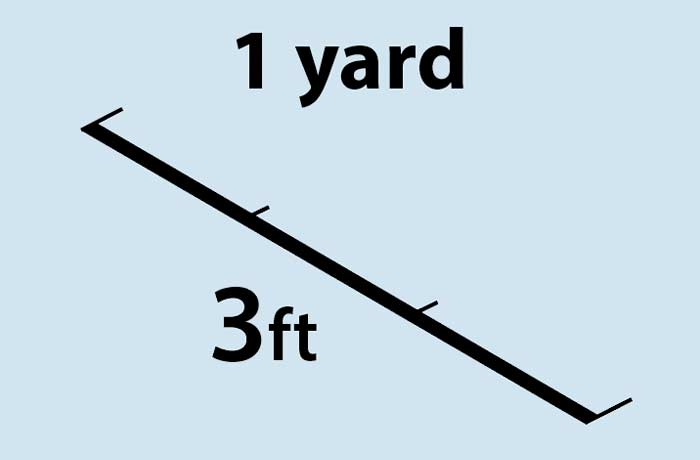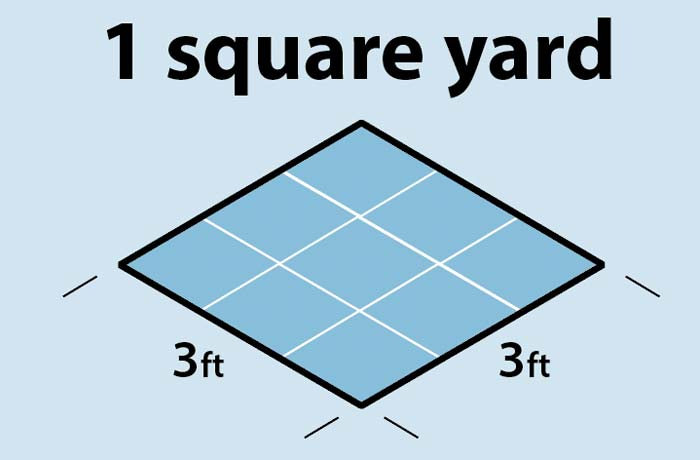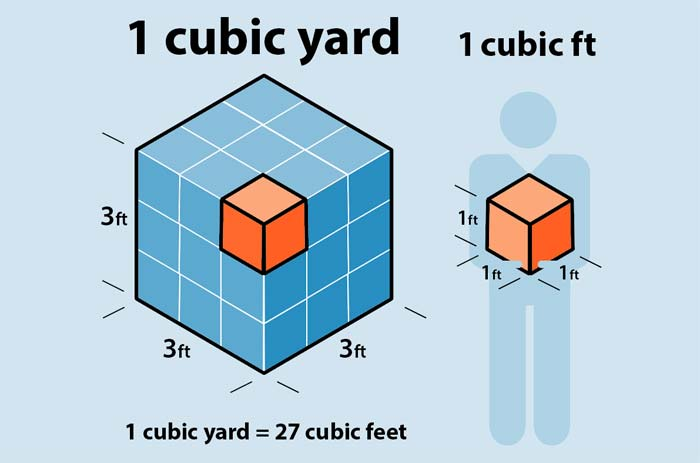# How Many Cubic Feet Are in a Yard?

| Last update: 28 July 2023

One cubic yard is equivalent to 27 cubic feet. This conversion is based on the fact that one yard is equal to 3 feet, with the three-dimensional cubic yard volume therefore being equal to 3 x 3 x 3 = 27.

You might find yourself embarking on a project in your house garden involving concrete, gravel, rock, mulch or other materials and wondering how to measure a cubic yard or how many cubic feet there are in a yard. Wonder no longer, here's what you need to know...

1 cubic yard = 27 cubic feet

## Explanation and diagrams

A foot is 12 inches long, making it exactly a third of a yard. So, if you draw a line on the ground with chalk and it's 1 yard in length, then it's 3 feet long.

### 1 yard = 3 feetIf you wanted to turn that one dimensional chalk line into a two dimensional area, you could use your line as one side of a square, a yard by a yard in measurement. How many feet are in a square yard? The two dimensions on the flat square (length and width) are each 3 feet long, so the sum is 3 x 3 = 9 square feet in a square yard.

### 1 square yard = 9 square feetNow what about if you wanted to turn that flat square into a three dimensional cube? You'd need it to be the same height up as it is across, making it a yard in every direction. So 3 x 3 x 3 (length x width x height) = 27 cubic feet in a cubic yard.

### 1 cubic yard = 27 cubic feetSo how many cubic feet are in a yard? Well, none if it's a chalk line on the ground, but if it's a cubic yard (sometimes just called a yard for measuring concrete or other materials), then it contains 27 cubic feet.

## How many cu ft bags of mulch are in a yard?

Bags of mulch commonly come in sizes from 1 cubic foot to 3 cubic feet, so you may be wondering how many 1, 2 or 3 cubic foot bags of mulch make up a yard (cubic).

• 1 cu ft: 27 bags of mulch in a cubic yard.
• 2 cu ft: 13.5 bags of mulch in a cubic yard.
• 3 cu ft: 9 bags of mulch in a cubic yard.

You can work out how much mulch you might need using this calculator.

### Before you go...

If you need to convert cubic feet to cubic yards then check out our cubic feet to cubic yards calculator. Alternatively, you can work out the capacity of an object or space using our cubic feet calculator.